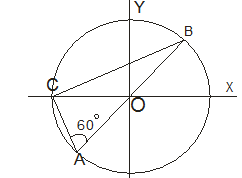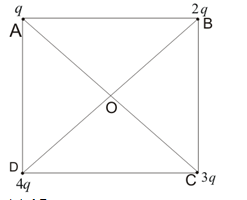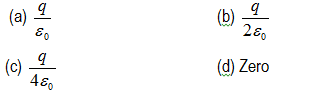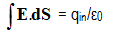Multiple Choice questions on Electric Charge for Jee Main and Advanced

In this page we have Multiple Choice questions on Electric Charge for Jee Main and Advanced . Hope you like them and do not forget to like , social share and comment at the end of the page.
Question 1
A charge of magnitude q is divided into two parts such that force between resulting two charges is maximum when separated through some distance r. The division of charges would be
(a) 3q/8 , 5q/8                                       (b) 2q/4 , 2q/4
(c) q/2 , q/2                                          (d) 3q/6 , 3q/6
Question 2
Consider a system of three charges q/3, q/3 and –2q/3 placed at points A, B and C respectively as shown in the figure. Take O to be the centre of the circle of radius R and angle CAB = 60º(a) The electric field at point O is q/8πε0R2                                 (b) The magnitude of the force between the charges at C and B is q2/54πε0R2
(c) The potential energy of the system is zero                 (d) The potential at point O is q/12πε0R
Question 3
Four charges q, 2q, 3q, 4q are placed at corners A, B, C and D of a square as shown below in the figure. The field at centre O of  square has the direction along(a) AB                                      (b) CB
(c) AC                                      (d) DB
Question 4
A point charge q is placed at geometrical centre of one of the face of a cube. The total flux through the cubical surface due to charge isQuestion 5
Two large metal sheets having surface charge density +σ and –σ are kept parallel to each other at a small separation distance d. The electric field at any point in the region between the plates is
(a) σ/ε0                                    (b) σ/2ε0
(c) 2σ/ε0                                   (d) σ/4ε0

Question 6
A rod lies along the x-axis with one end at the origin and other at x->∞ it caries a uniform charge λ C/m. Find the electric field at the point x=-a on the x-axis
(a)  -(λ/4πε0a)I                          (b)  -(λ/4πε0a2)i
(c)  (λ/4πε0a)I                           (d) (λ/4πε0a2)i

Question 7
Twelve charges of charge q are situated at the corners of the 12 sided polygon of side a. What is the net force on the charge Q at the centre
(a) Zero                        (b)  3qQ/πε0a2
(c)  qQ/πε0a2                            (d)  None of the above

Question 8
Two positive point charge are placed at the distance a apart have sum Q. What values of the charges , coulomb force between them is maximum
(a) q1=q1=Q/2                            (b) q1=3Q/4 ,q2=Q/4
(c) q1=5Q/6 ,q2=Q/6                   (d) Non of the above

Question 9
A metallic shell having inner radius R1 and outer radii R2 has a point charge Q kept inside the cavity. Electric field in the region R1 < r < R2 where r is the distance from the centre is given by
(a) depends on the value of r                              (b) Zero
(c) Constant and nonzero everywhere
(d) None of the above

Question 10
Consider two statements
A) The force with which two charges interact is not changed by the presence of the other charges
B) Electric force experienced by the charge particle due to number of fixed point charges is vector resultant of the forces experience due to individual charges
(a) A and B both are correct                              (b) A is correct only
(c) B is correct only                                           (d) A and B both are wrong
Question 11
A metallic solid sphere of radius R is given the charge Q. Which of the following statement is true then
(a) Electric field at points 0< r < R is zero                                   (b) Charge Q is on the outer surface of the sphere
(c) Electric field at r>R is given by Q/4πε0r2                                (d) Electric field is perpendicular to the surface of the sphere

Question 12.
A simple pendulum consists of a small sphere of mass and positive charge q is suspended by the string of length L. The pendulum is placed in the electric field of strength E directed vertically downwards. Which of the following is true
(a)Time period of oscillation=T=2π√L/(g+qE/m)                           (b)Time period of oscillation=T=2π√L/(g-qE/m)
(c) Tension in the string when the pendulum is at rest =mq+qE    (d)Tension in the string when the pendulum is at rest =mq-qE

Question 13
A rod lies on the x-axis with end and at x=-L and other end at x=L with uniform charge λ C/m. Which of the following is true
(a).Electric field at any point (0,y) on the y-axis is given by
E=(2kλL/y√(y2+L2))j
(b) For point on the Y-axis greater than y>>L
E=(2kλL/y2)j
(c) Electric field if L->∞
E=2kλ/y
(d) None of the above

Question 14
A particle of mass m and charge q is thrown horizontally with a velocity v from top of the building of height H. An electric field exists in the plane and it is horizontally away from the building
which of the following is true
(a) Range of the particle is greater than v√(2H/g)                        (b) Time of flight is √(2H/g)        (c) Path is parabolic                                                                  (d) None of the above
Question 15
At a point on the axis of an electric dipole
(a) Electric field is zero                                                              (b) Electric potential is zero
(c) Neither electric field nor electric potential is zero                    (d) Electric field is directed perpendicular to axis
Question 16
A point charge (Q) is located at the centre of a cube of edge length a, find the final electric flux over one face of the cube
a. Q/ε0
b. Q/6ε0
c. 6Q/ε0
d. none of the above
Question 17
Three point charges q + Q, q, q - Q are enclosed by the surface S. What the net flux crosses S
a. 3q
b. 2q
c. 3q - Q
d. can not be determine based on the data given in question
Question 18
Find the electric field inside the sphere which carries a charge density proportional to the distance from the origin
ρ = kr
a. ρ/ε0
b. ρr/ε0
c. ρr20
d. none of the above
Question 19
A point charge Q(C) is placed at the origin. Find the electric flux of which an area 4π m2 on a concentric spherical shell of radius R
a. Q/R2ε0
b. Q/ε0
c. Q/4R2ε0
d. none of the above
Question 20
As per Gauss lawa. This is valid for symmetrical surface only
b. E is the electric field to the charge inside the surface
c. Electric flux on the closed  surface due to outside charge is always zero
d. none of the above
Question 21
A uniform line charge with linear density λ lies along the y-axis. What flux crosses a spherical surface cantered at the origin with r = R
a. 2Rλ/ε0
b. Rλ/ε0
c. λ/ε0
d. none of the above

1. (c)
2. (b)
3. (b)
4. (b)
5. (a)
6. (a)
7. (a)
8. (a)
9. (b)
10. (a)
11. (a),(b),(c),(d)
12. (a),(c)
13. (a),(b),(c)
14. (a),(b)
15. (c)
16. (b)
17. (a)
18. (b)
19. (a)
20. (c)
21. (a)

Solutions